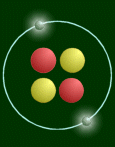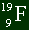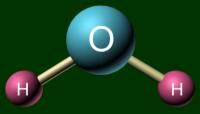MainTutorialsOrganic ChemistryPractice TestsOnline QuizzesReference ToolsAtoms:
By Takalah

Topics Covered

This section introduces some basic concepts of chemistry.

The Law of Multiple Proportions simply states: When two elements form a series of compounds, the ratios of the masses of the second element that combine with 1 gram of the first element can always be reduced to small whole numbers.

For those of you who are lost, it just means that only multiples of an amount of one element can react with a constant amount of another in a two element compound. For example, here is a table comparing three compounds of nitrogen and oxygen.

 Compound Amount of Nitrogen that reacts with 1 gram of oxygen Compound A 1.750 g Compound B .8750 g Compound C .4375 g

See the smallest number, .4375? It divides into the other numbers with no remainder. That is, B/C is 2, and A/C is 4. This just shows that molecules are made up of finite sized units, or atoms. You can't have 2.3 atoms combine with 1 atom. Same thing here.

There is no way to know from this data alone exactly the ratios of the molecules. You could take the base unit to be any one of the numbers. Let's do two examples.

Using .4375 and ratio 1N : 1O (Compound C):

Let's call .4375 the ratios of the molecules 1N : 1O. Since Compound B contains twice as much N, you could say that the ratio there is 2N : 1O. Since Compound A contains four times as much N as Compound C, you could say that the ratio is 4N : 1O.

Using .8750 and ratio 2N : 2O (Compound B):

Now you say that .8750 is your base number, and the ratio 2N : 2O. What will the ratio of Compound C be? You know that there is half as much Compound C as in Compound B, so just reduce the N by one-half; 1N : 2O. And since you know there is 4 times as much in Compound A, it's 4N : 2O. It's that easy.

As you can guess, there is an infinite number of situations that satisfy the table.

These are pretty straightforward and require no explanation. Although you don't use these on tests or anything, it's good stuff to know (not to mention our whole understanding of chemistry is based on this):

• Each element is made up of tiny particles called atoms.
• The atoms of a given element are identical; the atoms of different elements are different in some fundamental way or ways.
• Chemical compounds are formed when atoms combine with each other. A given compound always has the same relative numbers and types of atoms.
• Chemical reactions involve reorganization of the atoms--changes in the way they are bound together. The atoms themselves are not changed in a chemical reaction.

These ideas form the basis of what a lot of AP Chem is all about; chemical reactions. It's nothing more than breaking some bonds and making some bonds, to put the atoms in a different order to create new things.

An atom consists of a nucleus and electrons orbiting around it. The nucleus is made up of positively charged particles called protons, and neutral particles called neutrons. The electrons are negatively charged particles.

Here is a simplified picture:This atom has two protons (red), so it is a helium atom.

One thing that pictures like the above cannot express is the relative sizes of the nucleus and the atom. If the atom was really that big (diameter 108 pixels), then the nucleus would be 1/100th of a pixel big! Or on a bigger scale, if the nucleus was the size of a pea, then the atom would be as wide as a football field. Another thing is mass. Virtually all the mass is concentrated in the nucleus. The 'pea' would weigh 250 million tons!

There are two numbers to know about any atom. One is the atomic number, which is the number of protons (and electrons in a neutral atom), and the atomic mass number, which is how much the thing weighs. One proton's weight is close to one neutron's weight which is close to one. Electrons weigh virtually nothing when compared to the huge protons, so they don't affect the total mass. So the atomic mass number is simply the number of protons plus the number of electrons.

The notation of writing atoms is like this:The top number represents the mass number.

The bottom number represents the atomic number.

Some things to remember while solving these types of problems:

(Note: Problems of this simplicity will not be on the AP test, I guarantee that. We are not so lucky. But you will be tested in class on this stuff.)

• The bottom number is always the number of protons.
• The bottom number is also usually the number of electrons, but only in a neutral element. Ions come later.
• The number of neutrons is always the top number minus the bottom number. All you're doing is taking the total mass, taking away the portion that is due to the protons, and what you got left is the neutrons. Likewise, if you know number of neutrons and protons, to find this number all you got to do is add.

Example:

 Sample ProblemHere is a sample atom. How many electrons, protons, and neutrons does it got? Solution The bottom number is always the number of protons you have. So there are 9 protons. It's a neutral atom, by the fact that there are no plus or minus signs anywhere, so you can assume there are 9 electrons as well. There are 19 total nucleons (protons and neutrons) in the nucleus, and 9 are protons. So there are 10 neutrons. Easy.

Compounds are made up of two or more atoms held together in some way. Although I'll go way more in depth later, atoms can be bonded together by both sharing electrons or one taking electrons away from the other.

Those compounds that share form a covalent bond, and the resulting formation is a molecule. An example is water, H--O--H. Each H shares its electron with the O, so that the O has two shared electrons, completing its valence shell. Another is methane, CH4, which has each H sharing an electron with C, and C sharing a total 4 electrons with the H's.

A molecule acts as a unit by itself. It moves together. To represent a molecule, you can use either a ball-and-stick model or a space filling model. Examples of H2O are shown below.Ball-and-stick model Space filling model

As a general rule, a covalent bond results when two non-metals are put together.

Let's consider the case of NaCl. Chlorine has a higher affinity for electrons than sodium, and so it takes one away, shown by the following:

Cl + e- -> Cl-

This shows that the electron joins up with the chlorine, and becomes a negatively charged ion--also known as a anion. But where did this electron come from? From the sodium, of course:

Na -> Na+ + e-

This shows that the normal sodium atom gives off an electron--and becomes a positively charged ion in the process--also known as a cation.

Since these charges have opposite signs, they attract. The positive Na+ and the negative Cl- form a ionic solid, also a salt. (Keep in mind that a salt refers to any ionic solid, not just our example of table salt. Ions don't have to be just one atom; they can be polyatomic, or consisting of many atoms. Some examples are the anion C2H3O2- and the cation NH4+. A general rule, not to be followed strictly; if a compound has a metal and non metal in it, it's ionic. One of many exceptions is NH4Cl; I guess the best rule to follow is to look for those ions!

One of the most annoying things in chemistry is memorizing ions. Once every week, my class would have to memorize ten ions and have an Ion Quiz. Although you might forget them, it is a good idea to review them while preparing for the test. I have included an ion table in the Tables section.

I hated this part in Chem I. But it's not too bad. On a test, a question might be to mix dinitrogen pentoxide with something else. No problem, but what's that made of? This section will show you how to take a chemical structure and give a name to it, and vice versa.

Binary Ionic Compounds

These are just compounds made up of two different ions, like sodium chloride. All you do is: Put the name of the cation, followed by the name of the anion. That's it.

Example:

 Compound Name Ions KI Potassium Iodide K+, I- CaS Calcium Sulfide Ca2+, S2- MgO Magnesium Oxide Mg2+, O2- Li3N Lithium Nitride Li+, N3-

For single one-atom ion compounds such as above, you keep the name of the cation, and change the ending of the anion to -ide. Other examples are hydrogen => hydride, carbon => carbide, Fluorine => Fluoride, you get the idea.

One more thing to consider is the charge on the metal atom. If it can have only one possible value (such as +1 for sodium and +2 for magnesium above), then the normal name is fine. If the metal can form cations with different charges, like iron and tin, then you must put the Roman Numeral of it in parenthesis right after the name of the cation. Here are some examples:

 Metal Ion First half of compound name Fe2+ Iron (II) Fe3+ Iron (III) Pb2+ Lead (II) Pb4+ Lead (IV)

How do you know if to use roman numerals or not? Generally, if the metal is a transition metal (belonging to the three row skinny middle part of the periodic table up to the metalloids), then you need to use it. Some exceptions are aluminum (+3), zinc (+2), silver (+2), and cadmium (+2). Even though these are transition metals, they have only one possible ion. These metals and the metals in the first two columns need not use it, in fact, don't use them.

Another thing; mercury is really weird here. The two possible ions with mercury are Hg2+22+. The difference when writing this is that Mercury (II) for the first, and Mercury (I). Because there is a total of +2, and there are two.

Nothing to it. Before I get to the sample problems, there is one more important point to all this: Compounds don't have charge. So when you add up all the charge from the ions, they must equal zero. And if they don't, you must balance it. Note that in the first table, the first three ions, when charge is added up, give zero. The last one is different. It still must add up to zero, but note that N is -3, but Li is only +1. It's common sense that you need three Li atoms to cancel out the -3 in N, hence the formula.

Example:

 Give the name of these compounds: a.) CuCl b.) NaF c.) MnO2 d.) FeBr2 e.) Fe2O3 Answers By the rules, this is Copper Chloride. But copper can have different charges, so you must figure what the charge on this one is. Let's see: you know chloride can have only one value, and that's a -1. So if they must add up to zero, Cu must be +1. The answer is copper (I) chloride. This is sodium and fluorine, so the name is sodium fluoride. Sodium can only be +1, so there is no need for roman numerals. The name is Manganese Oxide, but a roman numeral is necessary, since it's a transition metal. In this case, manganese is a transition metal, so it needs a roman numeral. Let's start with oxygen: Each oxide ion has a charge of -2, and there are 2 of them. So the total charge due to oxide is -4. The single manganese ion must compensate for this, so its charge must be +4. The name is manganese (IV) oxide. Same here. It needs a roman numeral. Br is -1, and 2 of them, so Fe must be +2. Name is Iron (II) Bromide. e.This one's a little bit different, but the same concepts apply. You start with the oxygens. 3 of -2 ions make it a total of -6 charge due to oxide. So the two irons must compensate. If two of them must equal +6, each must be...+3!!! It should be no surprise. So the name of this is Iron (III) Oxide.

Polyatomic Ions

Many compounds don't have just one element for an ion. You can have multiple atoms of different types in an ion. One of the most common ones are oxyanions, an anion containing one atom and one or more oxygen atoms. They usually have a series associated with them, with the number of oxygen atoms varying. The naming system is simple; the thing you must memorize for each element is where the naming system begins.

Many compounds don't have just one element for an ion. You can have multiple atoms of different types in an ion. One of the most common ones are oxyanions, an anion containing one atom and one or more oxygen atoms. They usually have a series associated with them, with the number of oxygen atoms changing. The naming system is simple; the thing you must memorize for each element is where the naming system begins.

 Ion Name of Ion ClO- Hypochlorite ClO2- Chlorite ClO3- Chlorate ClO4- Perchlorate

Very easy stuff. Note that the charge is the same for all; it's not a coincidence. All members of a oxyanion series have the same charge. Other series are the phosphates and the sulfates. The trick is remembering for each series where it starts. Once you remember that, then it's easy to get the other names if you know the naming rules. Like for the chlorines, remembering that 3 oxygens is the chlorate form is all that you need. Then you know that if you add one oxy, it becomes perchlorate, take away one, then its chlorite, and so on.

The hardest part for some people, if any, when solving polyatomics is that an ion is a unit by itself. Like for example, when looking at Na2SO4, don't think of it as Na+, S2-, and O2-. That is wrong and will give you wrong answers. The real way to look at it is Na+ and SO42-. You can't break up that ion! And remember that when you need more than one polyatomic ion, use ()'s! If two hydroxides are needed to balance that 2+ calcium, use (OH)2 not OH2!

All other rules hold. You need roman numerals for transition metals, and the total charge must equal 0.

Covalent

The other binary compound is called covalent. If you have noticed, ionic compounds generally are metals joined to non metals, with exceptions. Covalent compounds are two non metals put together. This is by far the easiest way to name compounds. Just add the appropriate prefix to each of their names, along with the -ide suffix on the second element.

 Number of Atoms Prefix 1 mono- 2 di- 3 tri- 4 tetra- 5 penta- 6 hexa- 7 hepta- 8 octa- 9 nona- 10 deca-

One other little minor rule; if there is only one of the first element, don't use mono-. It's reserved only for the -ide part.

Examples:

CO2 => Carbon Dioxide

CO => Carbon Monoxide (note that it's not Monocarbon Monoxide, that sounds crazy! :)

N2O5 => Dinitrogen Pentoxide (change the a in 'penta' to o, awkward to have 'a' and 'o' next to each other)

SF6 => Sulfur Hexafluoride

Don't get this mixed up with the ionic stuff. You might be tempted to write Fe2O3 as Di-iron Trioxide because it's very easy, but that is very wrong. This prefix system is only for covalent compounds. Which is why students tend to like covalent compounds better than ionic compounds.

Using all that info above, you should be able to do the reverse of what we've been doing; that is, you should be able to supply a formula given a name. Let's try some:

Example:

 Find the formula for each chemical below. Sodium sulfate Vanadium (V) fluoride Mercury (I) chloride Tin (II) fluoride Cobalt (III) nitrate Answers The ions are Na+ and SO42-. But Na has only +1, and SO4 is -2. So you need two Na's to make up for that -2. The answer is Na2SO4. The ions are V5+ and F-. You know the +5 of vanadium by the (V). So you need 5 fluorines to make up for that +5. The answer is VF5. Mercury is the weird ion. Since it's (I), then the ion must be Hg22+. And since there is a +2, you need two Cl-'s to balance. The answer is Hg2Cl2. Tin is +2, so you need 2 F's to balance. Answer is SnF2. Cobalt is +3, and nitrate is -1. Need three nitrates to balance, so answer is Co(NO3)3.

That's it to this section. Go try the practice problems now!news | about us | contact us tutorials index | organic chemistry | practice tests | online quizzes | reference tools site copyright (c) 2002-2013 Learn ChemSubscribe to our low volume newsletter to receive up-to-date information about the CHEM SITE